# Provide the expected output AS IT WOULD APPEAR IN A JAVA PROGRAM as the result of a print statement. (i.e. Enter true or...

Provide the expected output AS IT WOULD APPEAR IN A JAVA PROGRAM as the result of a print statement.

(i.e. Enter true or false (all lower case letters). If you enter TRUE, F, True, etc these will be marked wrong).

Given the following declarations:

int x = 25;

int y = -4;

int z = 17;

boolean b = false;

What would be the output of the following Boolean expressions?

(x % 2 != 0) && b

b && !b

(z < x) == false

!(x / 2 == 13) || b || (z * 3 == 51)

x > y && y > z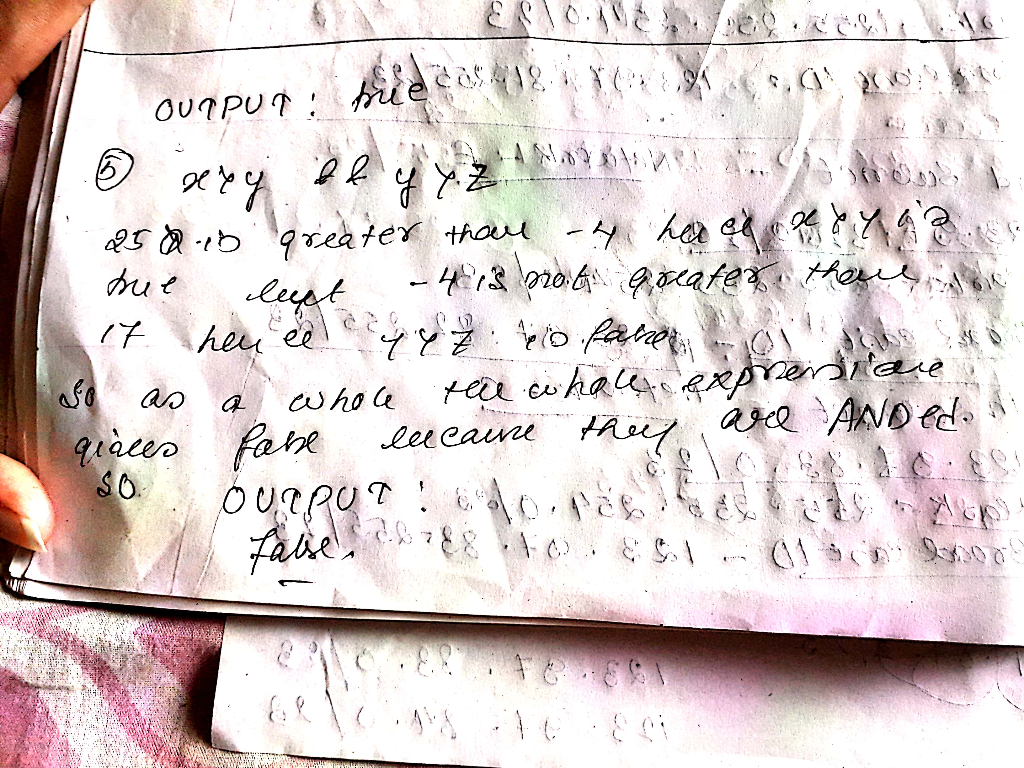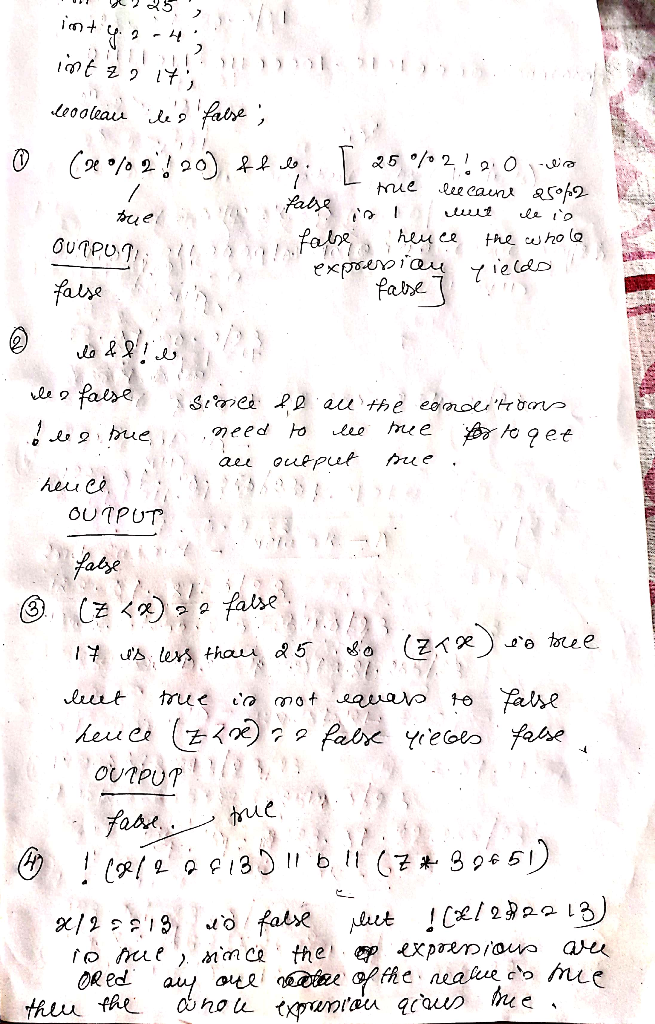##### Add Answer of: Provide the expected output AS IT WOULD APPEAR IN A JAVA PROGRAM as the result of a print statement. (i.e. Enter true or...
Similar Homework Help Questions
• ### Java password program error

I am receiving the following error that I can not figure out for the life of me. Java:80: class, interface, or enum expected } // end public class password^the program is as follows, :80: is the last lineimport java.util.Scanner;public class password{public static void main(String[] args){String s;Scanner in = new Scanner(System.in);System.out.print("Please enter your password");s=in.next();if(isGood(s))System.out.println("Password is valid");elseSystem.out.println("Password is invalid: must contain a minimum of" +"8 characters, 1 upper and lower case letter, 1 didget, and one of" +"the following special characters: !,...

• ### Please follow all the instructions and do all the parts (a-d)! Create a Java program which implem...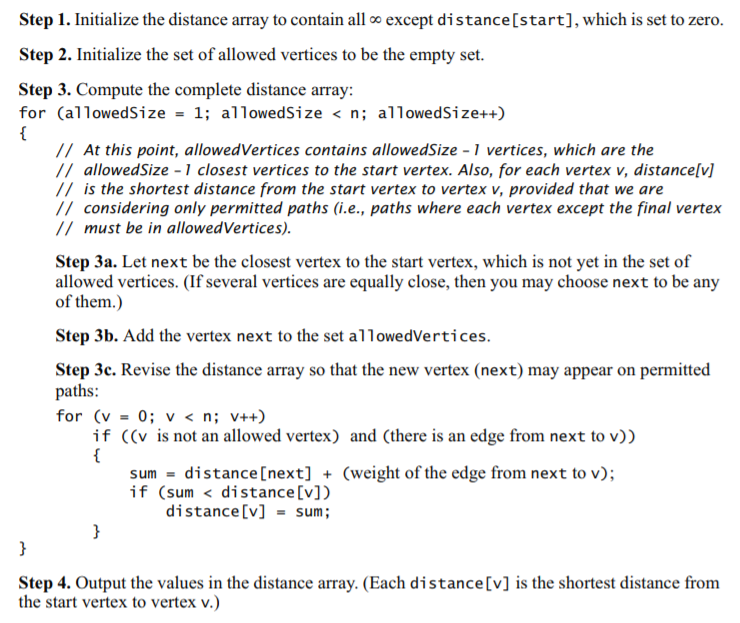Please follow all the instructions and do all the parts (a-d)! Create a Java program which implements Dijkstra’s shortest path algorithm according to the psueudocode below. Base the design on the weighted graph implementation used in Problem3.java (provided at the bottom). Your program should include the following features: a. A new method receives a starting vertex index and a target vertex index (e.g. 0 and 4). It computes the shortest distances to all vertexes using Dijkstra’s algorithm. The pseudocode is...

• ### java program error

I am recieving the following errors for the program listed below, can someone help out.java:112: variable declawed might not have been initializedjava:112: variable age might not have been initializedjava:115: variable name might not have been initializedonce compiled and working correctly I should get the program to print out and allow user input for each cat, then after input for all three cats has been inputted, theprogram is supposed to print out the following report:Names of cats >=3 that have clawsNameage/**********************************************************************************************************CatClawsProg.java**This...

• ### Java code Guessing Game Refinement. (The user thinks of a number and the program guesses it)...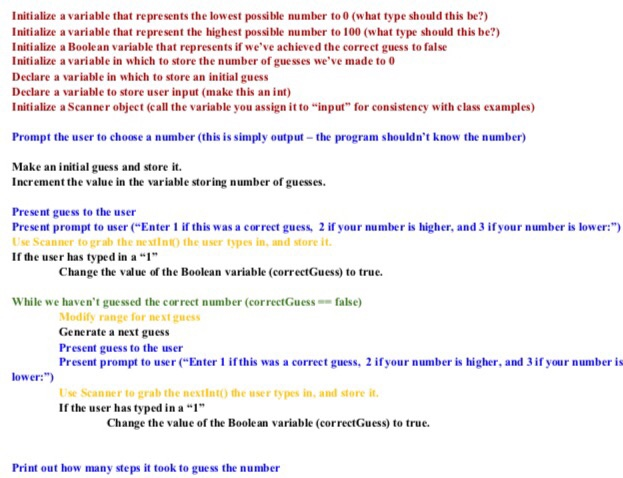Java code Guessing Game Refinement. (The user thinks of a number and the program guesses it) Program should be able to guess correctly in 7 tries or lower. Initialize a variable that represents the lowest possible number to 0 (what type should this be?) Initialize a variable that represent the highest possible number to 100 (what type should this be?) Initialize a Boolean variable that represents if we’ve achieved the correct guess to false Initialize a variable in which to...

• ### Suppose that you have the following variable declarations: int x = 10; int y = 15; int z = 20;

Suppose that you have the following variable declarations:int x = 10;int y = 15;int z = 20;Determine whether the following expressions are true or false.a. !(x < 10)b. x <= 5 || y > 15c. (x != 5) &&(y == z)d. x <= z &&(x + y >= z)7. What is the output of the following code fragment?int x = 100;int y = 200;if (x > 100 &&y <= 200)cout << x << " " << y << " "...

• ### JAVA String Program Problem #4 Assistance Neededx.øi5ave a JAVA string Project that I can not get to work.Below are the Requirements, Example to Solve the Program Project and the JAVA Code:Requirements:In this project, you will work with Java String. You are to write a program that does the following tasks:1) Ask the user for the name of the input file and the name of the output file2) Read a string from the input file (assume that the string is there)3)Determine its reverse string and write both...

• ### NEED HELP WITH THIS . Write a MARIE assembly language program that would simulate calling and exe...

NEED HELP WITH THIS . Write a MARIE assembly language program that would simulate calling and executing the following C function, as shown below: z = someFunction(a, b); where z, a and b are main( ) program variables, and the values stored in a and b are input by the user. The value stored in z will be output. Did your program execute correctly? This is the code for the function: int someFunction(int x, int y) { return 3 *...

• ### JAVA PROGRAM USING ECLIPSE. THE FIRST IMAGE IS THE INSTRUCTIONS, THE SECOND IS THE OUTPUT TEST...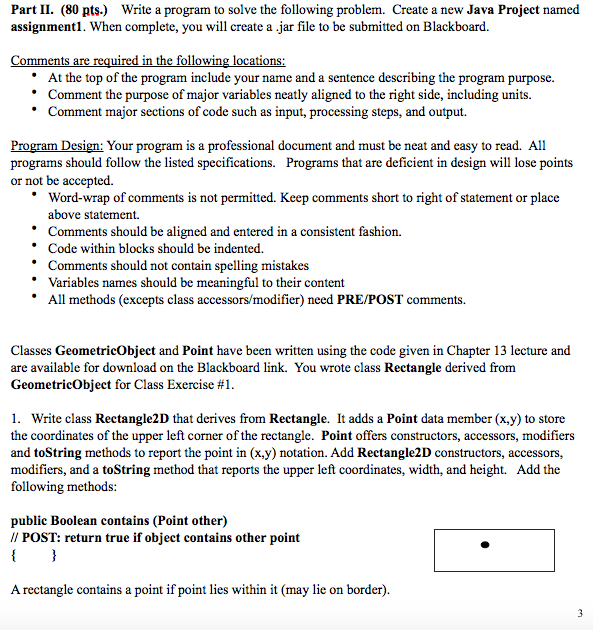JAVA PROGRAM USING ECLIPSE. THE FIRST IMAGE IS THE INSTRUCTIONS, THE SECOND IS THE OUTPUT TEST CASES(WHAT IM TRYING TO PRODUCE) BELOW THOSE IMAGES ARE THE .JAVA FILES THAT I HAVE CREATED. THESE ARE GeometircObject.Java,Point.java, and Tester.Java. I just need help making the Rectangle.java and Rectangle2D.java classes. GeometricObject.Java: public abstract class GeometricObject { private String color = "white"; // shape color private boolean filled; // fill status protected GeometricObject() { // POST: default shape is unfilled blue this.color = "blue";...

• ### x= Suppose you are building a program for teaching kids' math. Write a java program the...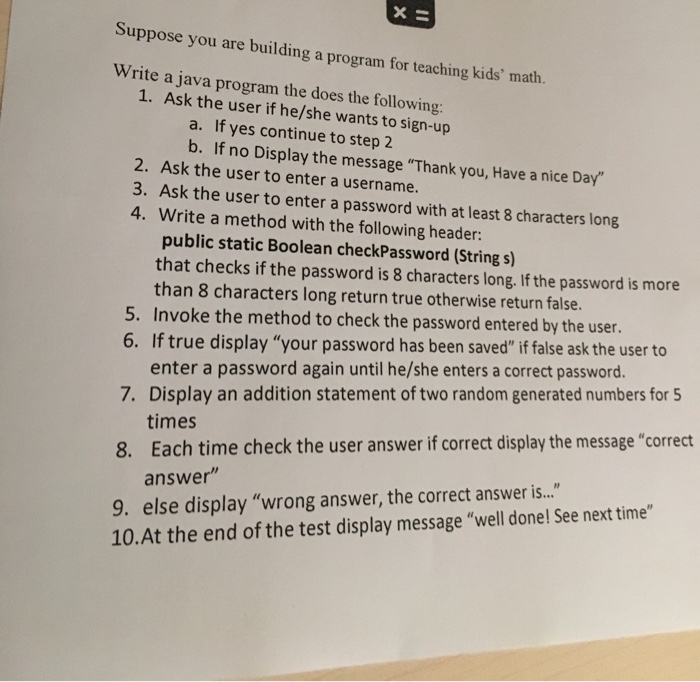x= Suppose you are building a program for teaching kids' math. Write a java program the does the following: 1. Ask the user if he/she wants to sign-up a. If yes continue to step 2 b. If no Display the message "Thank you, Have a nice Day 2. Ask the user to enter a username. 3. Ask the user to enter a password with at least 8 characters long 4. Write a method with the following header: public static Boolean...

• ### JAVA String Output Results Need !!!!Help!!!!!!!

x.øi5I use the input data “AaBb” to test the program, but I’m not getting the desired results highlighted in yellow. The output text file “tes1.txt” displays “AaBbbBaA 0 ( )” when I input the "AaBb" into the in.txt, and runthe program. The number of non-duplicate characters column should display “2 (A, B)”Below is Java String requirements, and the code:In this project, you will work with Java String. You are to write a program that does the following tasks:1) Ask the...

Need Online Homework Help?